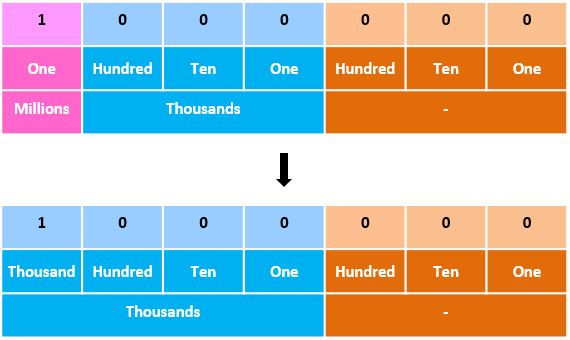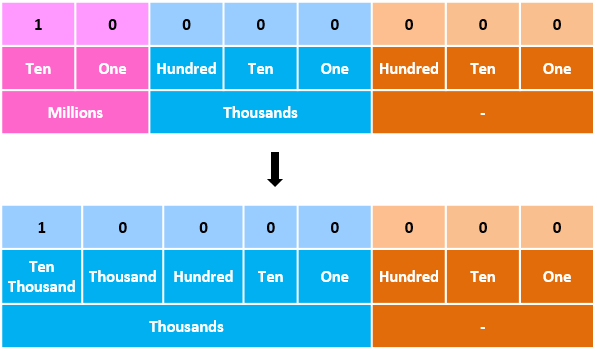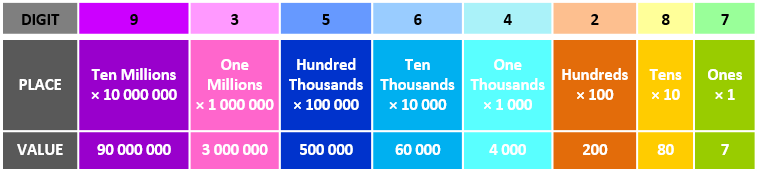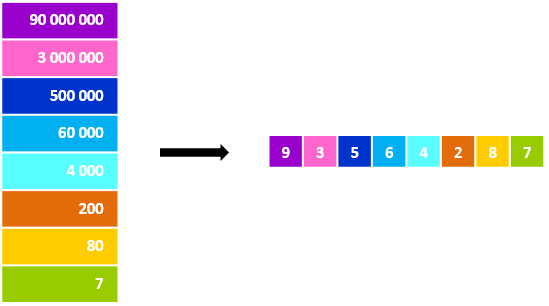## Place Values Upto 10 Million

Practice Unlimited Questions

#### 1. Understanding places and values of digits in a number

There are 10 different symbols that we use to generate numbers - 0, 1, 2, 3, 4, 5, 6, 7, 8 and 9.

Every number is a combination of these symbols or digits.

When we use these digits to make numbers, every digit is assigned a value based on the place in which it is positioned in the number.

Without places and values we would need a unique symbol to represent every possible number in the world!

#### 2. Finding the places and values of digits in a number

Let us take these two numbers: 478 and 3742. The digit 4 has a different value in each of these two numbers.

In 478, the digit 4 is in the hundreds place, so its value is 4 x 100 or 400 or four hundred.

In 48 236 951, the digit 4 is in the ten millions place, so its value is 4 x 10 000 000 or 40 000 000 or forty million.

So, the value of a digit is determined based on the position at which it is placed inside the number.

#### 3. How many thousands are there in 1 million?1 million  =  1 000 000
=  1 000 000
=  1000 000
=  1000 thousands
=  1000  times  thousand
=  1000  ×  1000

There are 1000 thousands in 1 million. There are one thousand thousands in 1 million.

#### 4. How many thousands are there in 10 million?10 million  =  10 000 000
=  10 000 000
=  10000 000
=  10000 thousands
=  10000  times  thousand
=  10000  ×  1000

There are 10000 thousands in 10 million. There are ten thousand thousands in 10 million.

#### 5. What is the value of each digit in 93 564 287?In 93 654 287, the digit
• 9 is in the ten millions place, so its value is 9 × 10 000 000, which is 90 000 000 or ninety million
• 3 is in the one millions place, so its value is 3 × 1 000 000, which is 3 000 000 or three million
• 5 is in the hundred thousands place, so its value is 5 × 100 000, which is 500 000 or five hundred thousand
• 6 is in the ten thousands place, so its value is 6 × 10 000, which is 60 000 or sixty thousand
• 4 is in the thousands place, so its value is 4 × 1 000, which is 4 000 or four thousand
• 2 is in the hundreds place, so its value is 2 × 100, which is 200 or two hundred
• 8 is in the tens place, so its value is 8 × 10, which is 80 or eighty
• 7 is in the ones place, so its value is 7 × 1, which is 7 or seven

Hence,